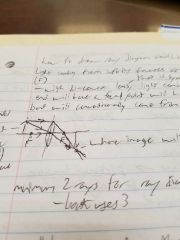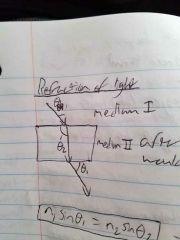• Shuffle
Toggle On
Toggle Off
• Alphabetize
Toggle On
Toggle Off
• Front First
Toggle On
Toggle Off
• Both Sides
Toggle On
Toggle Off
• Read
Toggle On
Toggle Off
Reading...
Front

## Card Range To Study

throughPlay buttonPlay buttonProgress

1/17

Click to flip

Use LEFT and RIGHT arrow keys to navigate between flashcards;

Use UP and DOWN arrow keys to flip the card;

H to show hint;

A reads text to speech;

### 17 Cards in this Set

• Front
• Back
 3 * 10^8 m/s is the speed is what in what medium? It is the speed of light in a vacuum and air. What is the formula for the refractive index of a medium? speed of light/speed of light in that medium = refractive index of a medium c/v=n What is the formula for reflection of light? Initial angle = reflective angle θi = θr n1sinθ1 = n2sinθ2 is what law? Snell's law What does a convex mirror do? It makes things smaller and is thick in the middle What is a concave mirror? It makes things larger that is thin in the middle. What is the drawing for image formation?What is do and di? do is the object distance. di is the image distance. Both are on either side of the focal point (x) at the center of the lens. What is the lens equation? 1/do + 1/di = 1/f What is the formula for magnification? m = hi/ho = -di/do What is hi and ho? hi is the image height. ho is the object height. What is a real image? An image that can be caught on a screen. What is it called if the image is too close to the lens that can't be caught. It is a virtual image. f = 8cm do = +24cm a. What is di?b. Is it real or virtual, straight or inverted? a. 1/di + 1/+24 = 1/8 m = -di/dob. Image is real, inverted, and diminished. What is the formula for velocity of light? V = fλ What makes an image straight up? What makes an image inverted? A positive magnitude means straight up, a negative magnitude means inverted. What is Snell's Law applied to?Refraction of light.